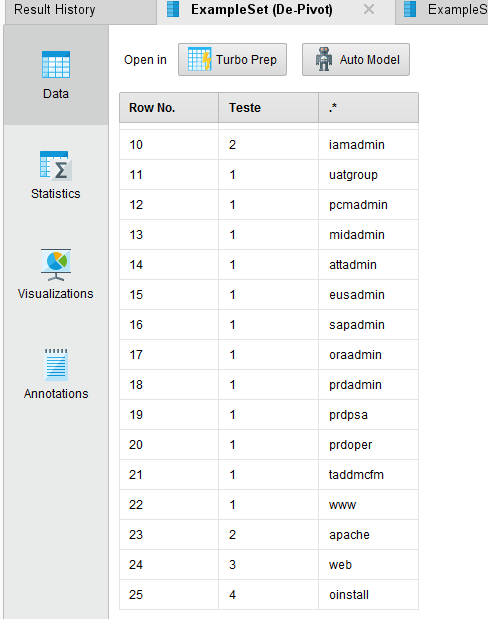# Split cell data into rows

Member Posts: 3Contributor I
Hello,

Is there a way to split data in a cell to rows, instead columns?

Example: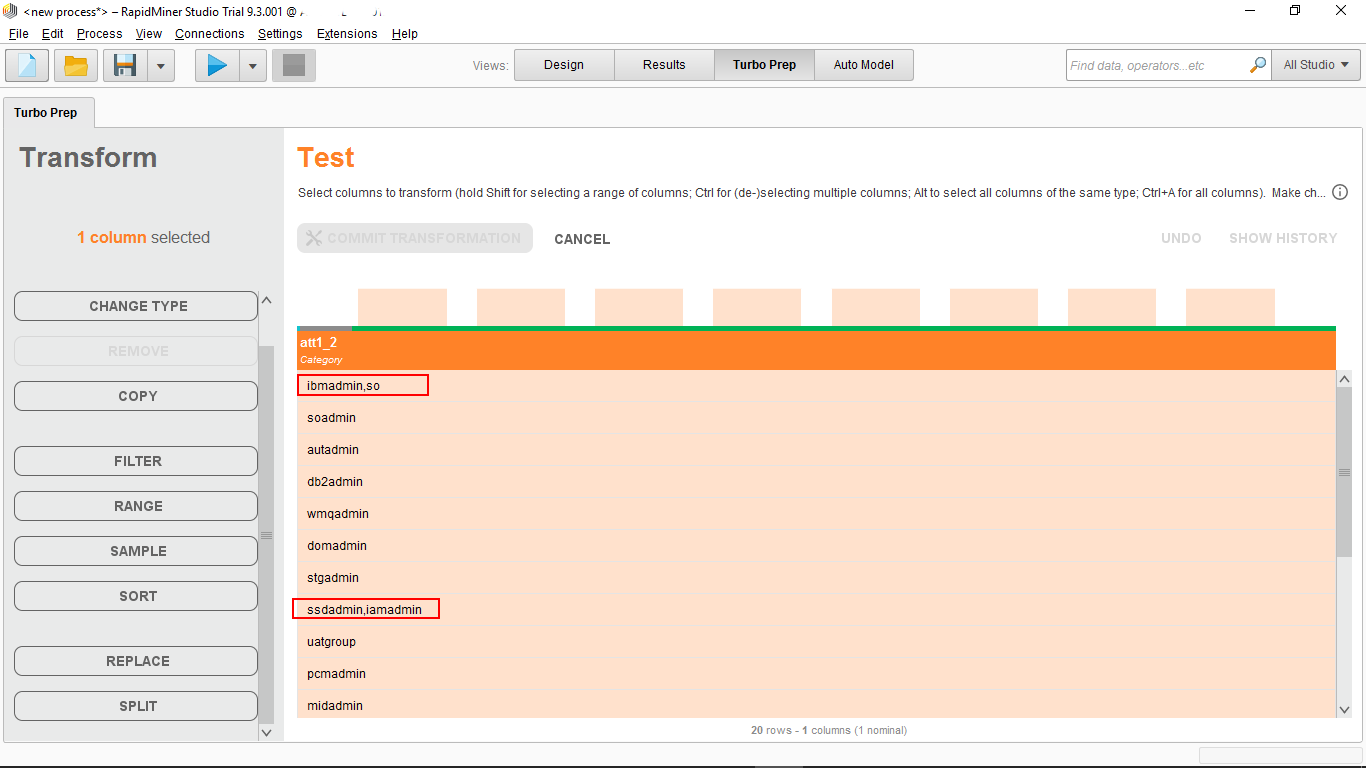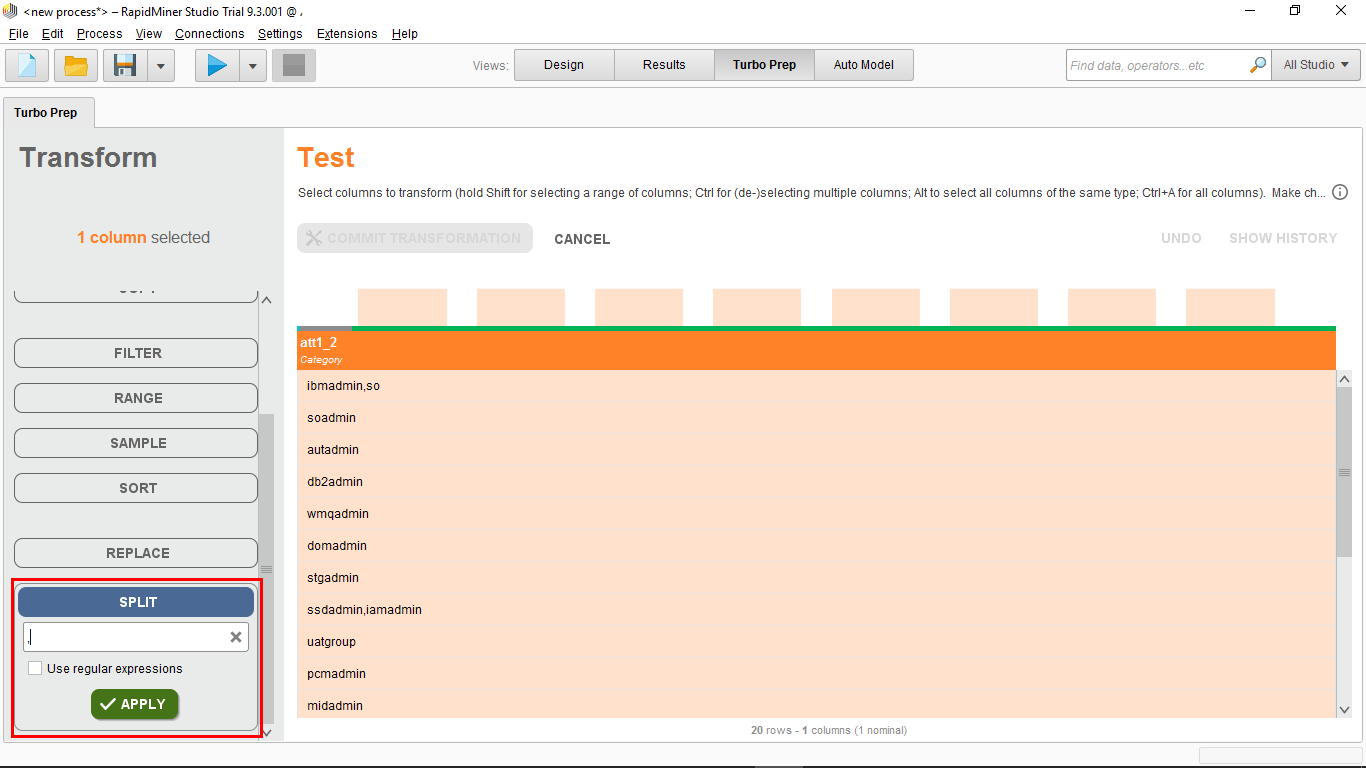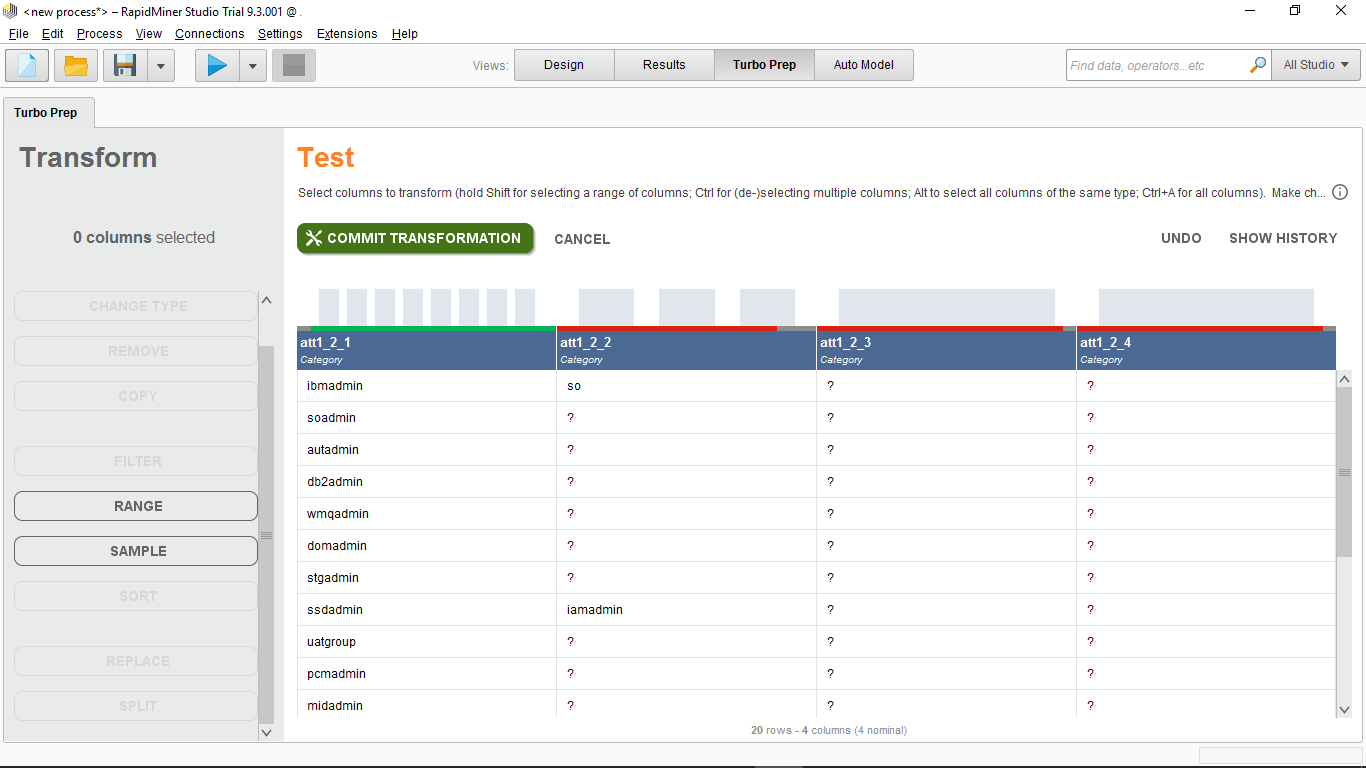What I really need now is the results one below the other, instead of columns....

Thank you!
Tagged:

• Administrator, Moderator, Employee, RapidMiner Certified Analyst, RapidMiner Certified Expert, University Professor Posts: 3,453RM Data Scientist
Puh,
i think not with TurboPrep: Are normal processes also fine?

Cheers,
Martin

- Sr. Director Data Solutions, Altair RapidMiner -
Dortmund, Germany
• Member Posts: 3Contributor I
Hi mschmitz,

Yes, is there a way to do it?
• Moderator, RapidMiner Certified Analyst, Member Posts: 1,195Unicorn
Hi @thiago_caixeta,

I propose an alternative method using a Python script  (Pandas library) to perform what you want to do :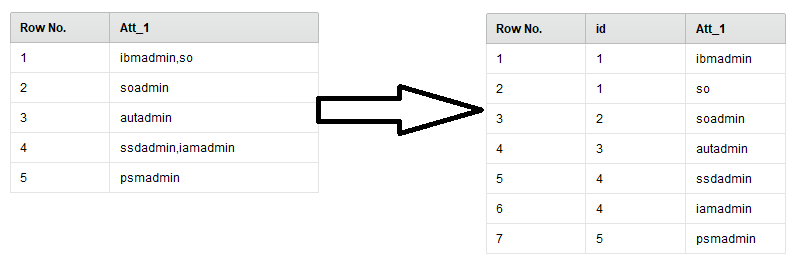To execute this process, you will need :
- to install Python on your computer.
- to install the Python Scripting extension from the Marketplace.

The process :

```<?xml version="1.0" encoding="UTF-8"?><process version="9.4.000-BETA">
<context>
<input/>
<output/>
<macros/>
</context>
<operator activated="true" class="process" compatibility="9.4.000-BETA" expanded="true" name="Process">
<parameter key="logverbosity" value="init"/>
<parameter key="random_seed" value="2001"/>
<parameter key="send_mail" value="never"/>
<parameter key="process_duration_for_mail" value="30"/>
<parameter key="encoding" value="SYSTEM"/>
<process expanded="true">
<operator activated="true" class="utility:create_exampleset" compatibility="9.4.000-BETA" expanded="true" height="68" name="Create ExampleSet" width="90" x="179" y="85">
<parameter key="generator_type" value="comma separated text"/>
<parameter key="number_of_examples" value="100"/>
<parameter key="use_stepsize" value="false"/>
<list key="function_descriptions"/>
<list key="numeric_series_configuration"/>
<list key="date_series_configuration"/>
<list key="date_series_configuration (interval)"/>
<parameter key="date_format" value="yyyy-MM-dd HH:mm:ss"/>
<parameter key="time_zone" value="SYSTEM"/>
<parameter key="column_separator" value=";"/>
<parameter key="parse_all_as_nominal" value="false"/>
<parameter key="decimal_point_character" value="."/>
<parameter key="trim_attribute_names" value="true"/>
</operator>
<operator activated="true" class="generate_id" compatibility="9.4.000-BETA" expanded="true" height="82" name="Generate ID" width="90" x="313" y="85">
<parameter key="create_nominal_ids" value="false"/>
<parameter key="offset" value="0"/>
</operator>
<operator activated="true" class="python_scripting:execute_python" compatibility="9.3.001" expanded="true" height="103" name="Execute Python" width="90" x="514" y="85">
<parameter key="script" value="import pandas as pd&#10;import numpy as np&#10;&#10;# rm_main is a mandatory function, &#10;# the number of arguments has to be the number of input ports (can be none)&#10;def rm_main(data):&#10;&#10;   new_data = pd.DataFrame(data.Att_1.str.split(',').tolist(), index=data.id).stack()&#10;   new_data = new_data.reset_index([0, 'id'])&#10;   new_data.columns = ['id','Att_1']&#10;   &#10;    &#10;   return new_data"/>
<parameter key="notebook_cell_tag_filter" value=""/>
<parameter key="use_default_python" value="true"/>
<parameter key="package_manager" value="conda (anaconda)"/>
</operator>
<connect from_op="Create ExampleSet" from_port="output" to_op="Generate ID" to_port="example set input"/>
<connect from_op="Generate ID" from_port="example set output" to_op="Execute Python" to_port="input 1"/>
<connect from_op="Execute Python" from_port="output 1" to_port="result 1"/>
<portSpacing port="source_input 1" spacing="0"/>
<portSpacing port="sink_result 1" spacing="0"/>
<portSpacing port="sink_result 2" spacing="0"/>
</process>
</operator>
</process>
```

Hope this helps,

Regards,

Lionel

• Member Posts: 3Contributor I
Worked fine!!!!

Thank you very much for your help!!